July 31, 2016

Question 1

(a)(b)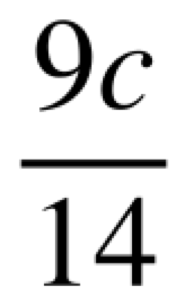(c)(d)(e)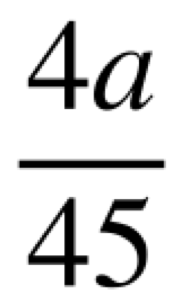(f)(g)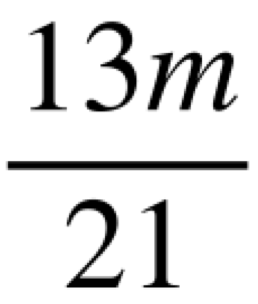(h)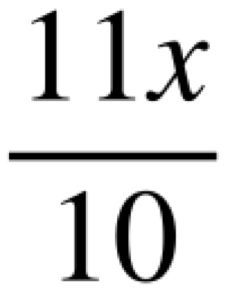(i)(j)(k)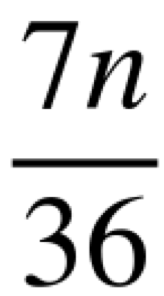(l)Question 2

(a)(b)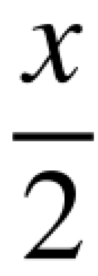(c)(d)(e)(f)(g)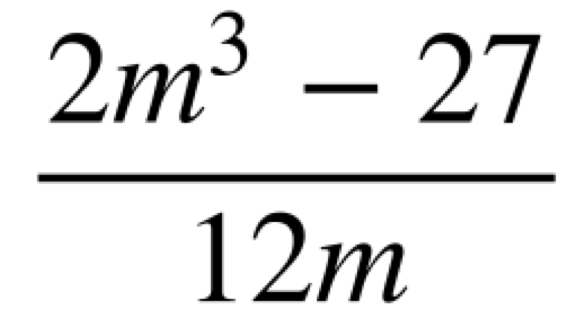(h)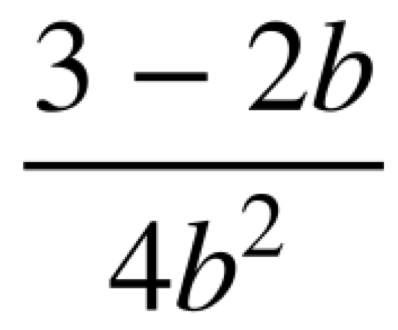(i)(j)(k)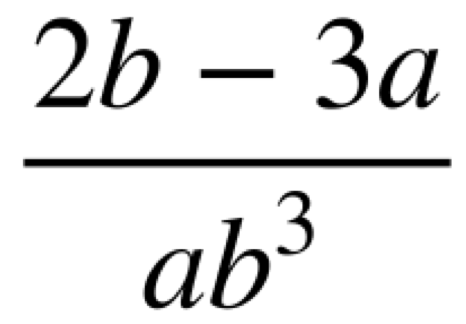(l)Question 3

(a)(b)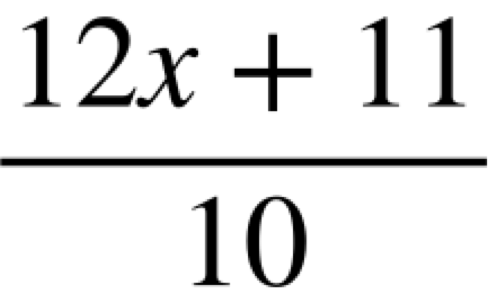(c)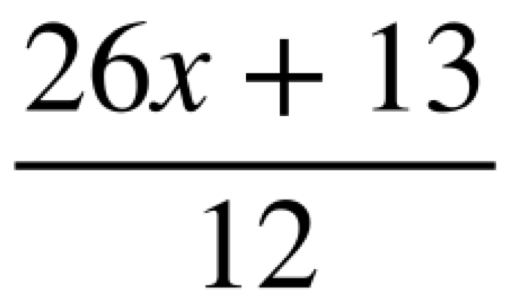(d)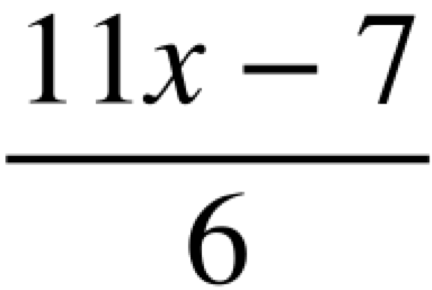(e)(f)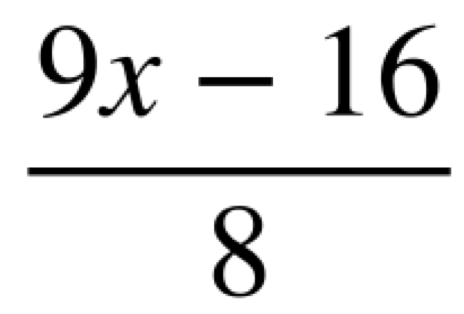(g)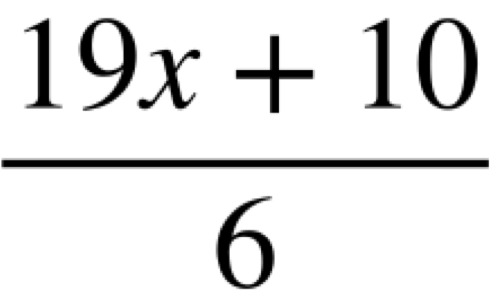(h)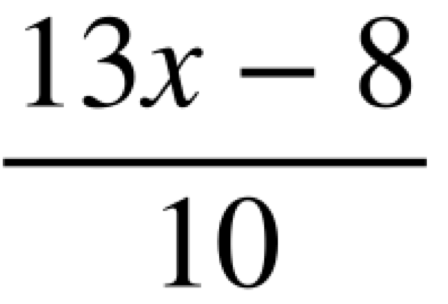(i)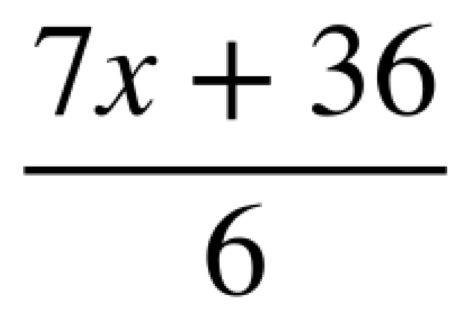(j)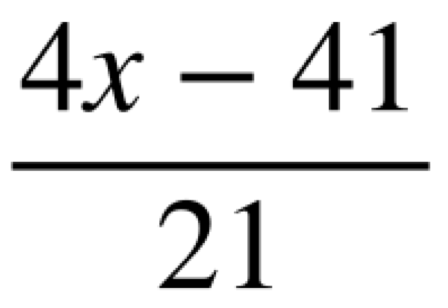(k)(l)Question 4

(a)(b)(c)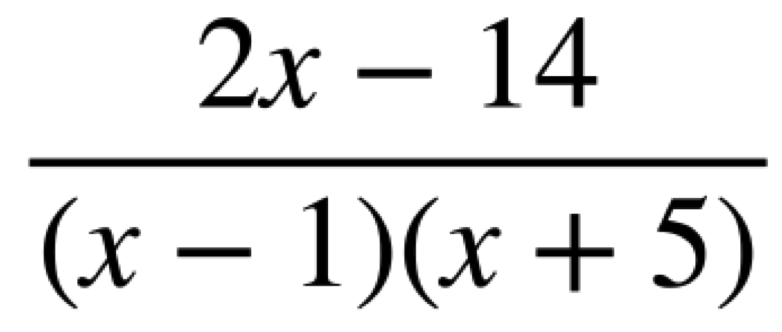(d)(e)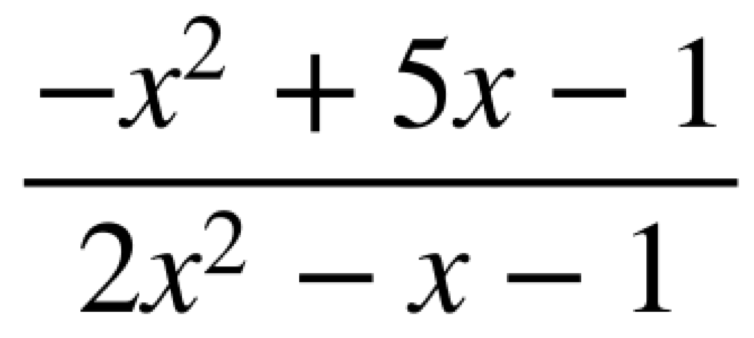(f)(g)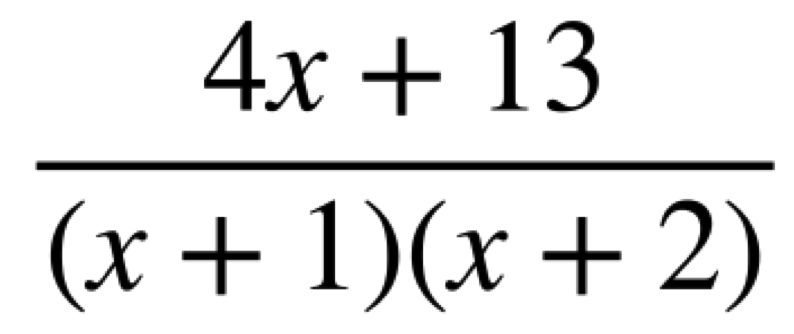(h)Question 5

(a)  x = 7

(b)  x = 19

(c)  x = 5.5

(d)  x = -6.25

(e)  x = -2.5

Question 6

(a)  x = 0   or   x = 3

(b)  x = 3.5  or   x = 5

(c)  x = 3   or    x = 6

(d)  x = 1    or   x = 2

(e)  x = -1   or  x = 4

(f)  x = -5    or  x = 7